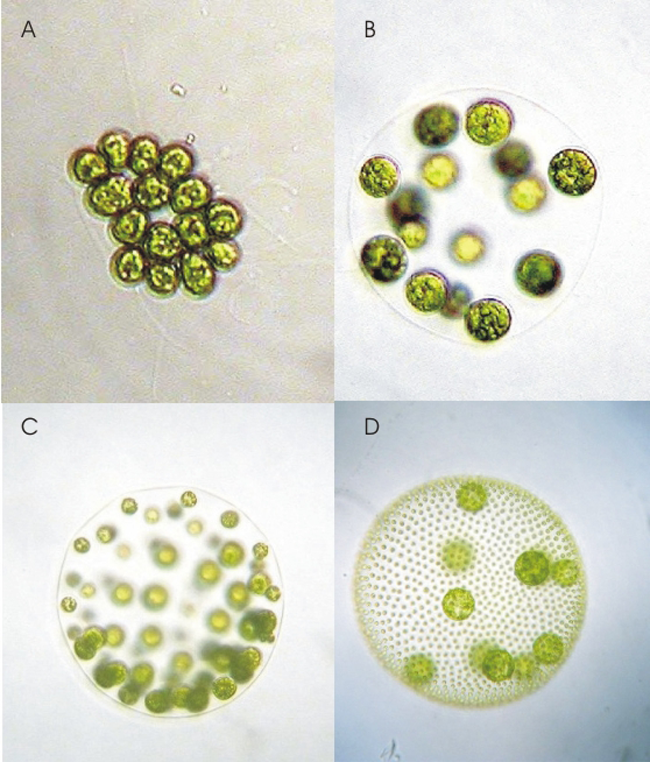# Instinct to thrive

Probability Level 5Let me introduce you to the unicellular organism code-named as:$Riru$. A cell of $Riru$, at the end of each day, fragments into $r$ daughter cells, where $1\le r\le 4$. The probability of $r$ taking a certain numerical value, is directly proportional to that value itself. Al the beginning of day $1$, one cell of $Riru$ was present in an isolated habitat, with plenty of resources. Find the average $Riru$ population at the beginning of day $21$.

×